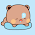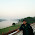Friday, May 7, 2010

Maximum Matching (Hopcroft)

Hopcroft-Karp is one of the fastest algorithm that finds the maximum cardinality matching on a bipartite graph. It has the best known worst case time complexity. More details can be found here [courtesy of Wikipedia].

C++ Source Code:

`#define MAX 100001#define NIL 0#define INF (1<<28)vector< int > G[MAX];int n, m, match[MAX], dist[MAX];// n: number of nodes on left side, nodes are numbered 1 to n// m: number of nodes on right side, nodes are numbered n+1 to n+m// G = NIL ∪ G1[G[1---n]] ∪ G2[G[n+1---n+m]]bool bfs() {    int i, u, v, len;    queue< int > Q;    for(i=1; i<=n; i++) {        if(match[i]==NIL) {            dist[i] = 0;            Q.push(i);        }        else dist[i] = INF;    }    dist[NIL] = INF;    while(!Q.empty()) {        u = Q.front(); Q.pop();        if(u!=NIL) {            len = G[u].size();            for(i=0; i<len; i++) {                v = G[u][i];                if(dist[match[v]]==INF) {                    dist[match[v]] = dist[u] + 1;                    Q.push(match[v]);                }            }        }    }    return (dist[NIL]!=INF);}bool dfs(int u) {    int i, v, len;    if(u!=NIL) {        len = G[u].size();        for(i=0; i<len; i++) {            v = G[u][i];            if(dist[match[v]]==dist[u]+1) {                if(dfs(match[v])) {                    match[v] = u;                    match[u] = v;                    return true;                }            }        }        dist[u] = INF;        return false;    }    return true;}int hopcroft_karp() {    int matching = 0, i;    // match[] is assumed NIL for all vertex in G    while(bfs())        for(i=1; i<=n; i++)            if(match[i]==NIL && dfs(i))                matching++;    return matching;}`

The implementation is quite straight forward as the algorithm on Wikipedia page. I am looking for some optimizations.

1.This works? Do you have a testing program also? please post it because i just can't figure where the problem is. Maybe at me :)

2.hello. i would like to know what the input data is. thank you in advance!

3.Hi ,i'm from Vietnam.

4.Yes this works, I think. I used it in a problem:
MATCHING @ SPOJ
https://www.spoj.pl/problems/MATCHING

Sorry I don't have any testing program yet.

I am learning matching for only a few days, so may be this is not a source which will solve any problem of matching, but I think it could be modified accordingly.

Thanks :)

5.But what is NIL node ? Is it an extra node that must be added in the graph before we launch the algorithm ? In this case how are NIL node edges defined ? NIL node has no edges ?

6.NIL node is nothing but just a reference node, it is added to the graph prior to applying the algorithm. As you see, it has no particular use in the algorithm, except used as a marking item, i.e. whether some node has some match or not. G is left blank (no edge) and NIL has the value 0, so, we are calling Node with number 0 to be a NIL node. I tried to stick to the original algorithm presented at wikipedia, the actual implementations are more straight forward, but those are specific problem dependent.

7.I tried the sample input

5 4 6
5 2
1 2
4 3
3 1
2 2
4 4

for the following main():

int main() {
int A, B;

scanf("%d %d %d", &N, &M, &P);
while (P--) {
scanf("%d %d", &A, &B);
G[A].push_back(B);

}

printf("%d\n", hopcroft_karp());

return 0;
}

It gives 2, correct answer is 3.

What's wrong with the main? or what's missing?

8.I wrote the following main() to work with your code:

int main() {
int A, B, P;

scanf("%d %d %d", &N, &M, &P);
while (P--) {
scanf("%d %d", &A, &B);
G[A].push_back(B);

}

printf("%d\n", hopcroft_karp());

return 0;
}

It gives 2, correct answer is 3.

What's wrong with the main() function? or what's missing?

9.Sorry, can't say without reading the whole code. However, this implementation is well tested as well. Although the right portion of the graph is not necessary.

10.Yes, it's my mistake, in the main function. Thanks.

11.12.could you please give me some more test case...thanks.. :)

13.I made such a program based on Your code: http://ideone.com/nlschW and as You can see it prints 2 instead of 3. Did I make a mistake or Your code is incorrect? I wouldn't ask but having copied exactly Your code and main from the comment I got 2 as well.

1.I don't understand your input. Would you explain your input a bit? This code only works for bipartite graphs.

2.Of course. In the first line we have size of sets A and B and number of edges t. In next t lines we have two numbers: x y which shows that x from set A and y from set B are connected. It is then a bipartile graph (only two sets). Here's an illustration: http://imageshack.us/photo/my-images/809/graphus.png

3.I get it now. My fault. The input was in incorrect format. Indexes from first set cannot be the same as these from the second one. So I just have to change tab[a].push_back(b) to tab[a].push_back(b+n) and it works. Thank You!

4.The pleasure is mine. Here is the link of my code library, all are tested codes. If you want to try any of them, make sure to read the comments, they will explain usage. http://zobayer2009.wordpress.com/code/

5.Thank You! Your algorithm for calculating minimumExpression is so much faster than my Duval's algorithm (also linear,though)! Added to bookmarks. :-)

6.All credit goes to the man who invented it. I just implemented, I don't deserve any credit for that. :)
Thanks for visiting :)

7.Sorry for spamming but I forgot to mention: there is a builtin for popcount so You can omit this several-line function. More:
Hope You find it useful.
// There are other interesting tricks on Ideone's status which You may want to check out.

8.No problem, and thanks for sharing. I actually never used popcount function. I have seen people use internal popcount in codeforces and other judges, but I always write them manually.
Book marked your link, I will surely check out after I complete the task at hand. Thanks again :)

14.hey. plz tell me how get matched nodes. this for above test we got there are matches. should we get matched node.
whether
3 1
2 2
4 4
are the matched three. or not plz explain.

1.make sure your input follows this:
// n: number of nodes on left side, nodes are numbered 1 to n
// m: number of nodes on right side, nodes are numbered n+1 to n+m
// G = NIL ∪ G1[G[1---n]] ∪ G2[G[n+1---n+m]]
then the match[] array should contain the matching nodes for 1 to n.

2.Thanks.It is one dimentional array and print only some values of set m nodes. how can we say that which nodes of n was matched with the solution

3.can we use it for weighted graphs. My problem is finding stable marriage couple using graph theory. can we use your code to solve the problem. Give me some instructions

15.match[u] = v means u is matched with node v.

1.2.This comment has been removed by the author.

3.Oh, I didn't notice that comment. There are straight forward greedy approaches to solve stable marriage problems. However, if you still want to do that using bipartite matching, this piece of code is not going to help. As you see, the path augmenting algorithm is BFS, and in stable marriage, you need to consider weights. So, you can use the general method of finding bipartite matching using maxflow algorithm where you can easily handle / maximize / minimize flow and costs. I assumed you know how bipartite matching problems can be solved using maxflow

4.This comment has been removed by the author.

16.Hi, I'm new in c++.
What should be the input of G looks like? I for the vector.
when 1 is matched to 3, is it means that G.push_back(3)?

besides, should I also input the match[] array?what should I put inside?

1.Nope, match[] array is the output array.
If you have N nodes in left side, and nodes are marked from 1 to N for left side and 1 to M for right side, then for this particular implementation, you have to something like:
input edge(left, right);
right = right + N;
G[left].push_back(right);
G[right].push_back(left); // not always necessary

and you get the match[] array after running hopcroft, if you find match[u] = v, it means, u is matched with right side node v-N.
Here is a sample main function:

int main() {
int i, u, v;
scanf("%d%d%d", &n, &m, &p);
for(i=0; i<p; i++) {
scanf("%d%d", &u, &v);
// u in G1, v in G2
v += n;
G[u].push_back(v);
G[v].push_back(u);
}
printf("%d\n", hopcroft_karp());
return 0;
}

2.This program will run for how long? I tested with the sample
http://www.spoj.com/problems/MATCHING/
but it seems to run so long.

3.The program ran for hours could not stop. What happened?

4.This comment has been removed by the author.

5.Sorry for late answer. Actually this is my solution for MATCHING
My program is not fast, but it was able to get AC in 3.16 seconds consuming 7.1 mega bytes memory.
This is my spoj profile: Zobayer

17.@Zobayer : You can make it more faster by checking the condition for dist[NIL]!=INF inside the bfs.It will make faster (My code on writing that line made it pass in only 2.06 sec whereas the code in which i wrote it at the end passed in 3.31 sec)

18.@Zobayer : Do you have any implementation for min cost maximum matching in a bipartite graph ? I have been following your blog since long.. So it would be great if you could upload a blog on the same.

1.I don't know direct algorithms like Hungarian to solve mincost matching, but this problem can easily be mapped to a mincost flow, (where your cost is the same thing, with a capacity 1 for each edge) as in your case it is a bipartite graph, it will run really fast. I will post it as soon as possible :)

19.Why are you checking (dist[NIL]!=INF) and returning its value?
even when you are never modifying dist[NIL].

What does BFS return??

20.why do we need to check dis[match[v]]==dis[u]+1 in dfs() function.How its helping and what if i dont check.
I am not understanding

21.how do you use "return (dist[NIL]!=INF);" in bfs?

1.its a boolean statement. it returns true if dist[NIL] is not INF, that is, it was updated at least once. otherwise returns false.

22.Incase someone hasn't mentioned it, you only need adj[u].push_back[v]. The graph is essentially for the vertices to the left and you only need to add the edges corresponding from the left to right.

I have solved MATCHING in 0.12 seconds, faster than most. Here is the code is used: https://github.com/foreverbell/acm-icpc-cheat-sheet/blob/master/code/hopcroft-karp.cpp

1.Thanks for mentioning it. Yes, I don't know why I added it back then. It's a long time ago. The algorithm does not traverse them anyway. Without any optimization, my code passes in 0.28 seconds using simple scanf and printf IO. I think its pretty good timing too.

I had a plan to optimize these old codes and repost better ones (if possible) here, but life got in the way :(

2.Btw, I have checked your code, its very clean. But it can be made even faster I believe (could be wrong though). Don't you think the loop in dfs() can be splitted? with one for the -1 flags and the other where it calls dfs.
I have an example here. https://zobayer2009.wordpress.com/code/#bpm_dfs
Thanks for visiting the blog :)

23.In some problems you may need an additional dfs for bicoloring to decide the value of n and m. More precisely, you may often do some extra work to decide which nodes go to left and which nodes go to right and you may need extra bfs/dfs for bicoloring. There is a pretty similar implementation of the above algorithm which takes care of this problem. Also, it encapsulates all methods and classes used by the algorithm in a separate class which makes it a little simpler to use the algorithm as template.
https://pastebin.com/pvPRuGXr
Tested on
http://codeforces.com/contest/468/problem/B
Happy Coding

24.Sorry brother, I was wrong. Please ignore my previous message.